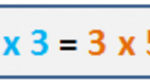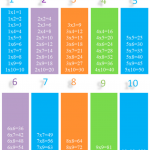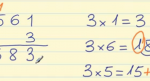Smartick is an advanced online program that teaches kids math and coding in only 15 min. a day

# I want to learn about: Mutiplication

Content about multiplication in elementary mathematics. Exercises, tutorials, problems, and teaching resources for elementary multiplication. Here you will find posts about multiplication to make learning mathematics easier and more fun.

Nov03

## Practice Applying the Distributive Property of Multiplication with ExamplesIn this post, you’ll learn another simpler, quicker and more efficient way to multiply these kinds of problems by applying the distributive property in multiplication. Surely, you all know how to calculate this multiplication problem, 23 x 4, using the multiplication algorithm: Or maybe you know how to do it mentally. 23 times 4 is […]

Oct04

## Commutative Property in Multiplication and AdditionIn this post, we’re going to see what the commutative property is all about. Example Charles and George learned how to calculate the area of a rectangle in math class by using the base by height formula. Each of them was given a problem using the same rectangle, asking them to calculate the area. Charles […]

Sep01

## Quick Multiplication Tricks for Fast and Effective MathDid you know that we can save a lot of time by multiplying? Every day we are faced with problems we can solve much faster with multiplication. For example: If we want to know how many people attended the football match… …we can count them one by one, but it would take us a lot of time, and it […]

Aug22

## How to Solve Multiplication Word Problems

Are you good at solving word problems? Solving word problems is a very important part of mathematics because with them you understand and practice what you have learned to do (adding, subtracting, multiplying…) Today we are going to look at multiplication word problems: how we recognize them, what to do to solve them…all of which […]

Aug18

## Learn the Different Properties of MultiplicationIn this post, we will do a review of the properties of multiplication and look at some of its applications. We begin with a brief summary of the properties of multiplication: Properties of Multiplication Commutative Property: The order of the factors does not change the product. 3 x 5 = 5 x 3 Associative Property: […]

Aug03

## The Distributive Property of Multiplication

Hello! We’re back again. Today we will look at one of the properties of multiplication: the distributive property of multiplication. As you may know, multiplication has different properties, let’s review them: Commutative property Associative property Identity Property Distributive property Let’s focus on the distributive property of multiplication The distributive property of multiplication states that when […]

Jul27

## Mental Math: Multiplying by 11

In this post, we are going to learn a mental math strategy for multiplying by 11 using a simple method. Mental Math Strategy 1: Multiplying a 2-digit number by 11 It’s only a matter of adding the number’s two digits and then putting the result in between the two numbers. Let’s take a look at some […]